Function Repository Resource:

# BinarySearch

Find the position of a target value within a sorted array

Contributed by: Wolfram Staff
 ResourceFunction["BinarySearch"][v,val] gives the position of the last element of a sorted numeric vector v such that the element ei≤val.

## Details

In ResourceFunction["BinarySearch"][v,val], v can have repeated elements.
The vector v must have at least two elements.
ResourceFunction["BinarySearch"] returns 0 if all elements ei>val.
For the sake of performance, ResourceFunction["BinarySearch"] does not check if the input list v is sorted, and can give an erroneous result for unordered lists.

## Examples

### Basic Examples (1)

Find the position of the last element that is not greater than 6 in a list of numbers in ascending order:

 In:=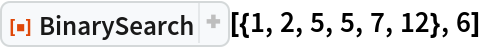Out=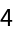### Properties and Relations (2)

BinarySearch[v,val] returns 0 when all elements of v are greater than the val:

 In:=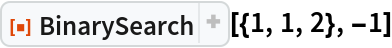Out=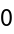BinarySearch can be considerably faster for packed arrays:

 In:=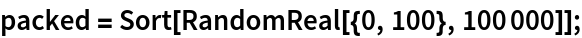In:=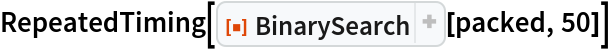Out=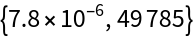In:=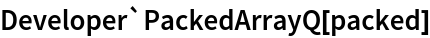Out=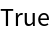In:=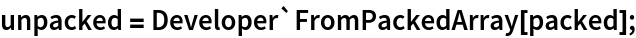In:=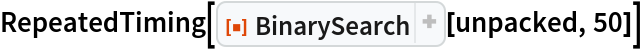Out=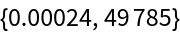### Possible Issues (2)

BinarySearch can give an erroneous result if the input vector is not sorted:

 In:=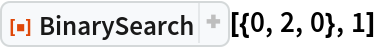Out=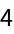Use LengthWhile to find the position of first element less than 1 in an unsorted list:

 In:=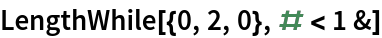Out=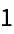## Version History

• 1.0.1 – 28 June 2021
• 1.0.0 – 09 March 2020